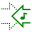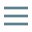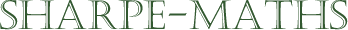# Glossary of terms

## M

•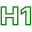### Multiplication

•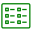Multiplication is a shorthand notation in arithmetic, to avoid lengthy addition problems. For example, $3+3+3+3+3+3+3+3+3+3+3+3+3+3$ can be written as $3 \times 14$, which tells us that $14$ $3$s are to be added together. As the numbers involved get bigger, the shorthand becomes more and more useful.

•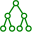$5 \times 8 = 40$
•